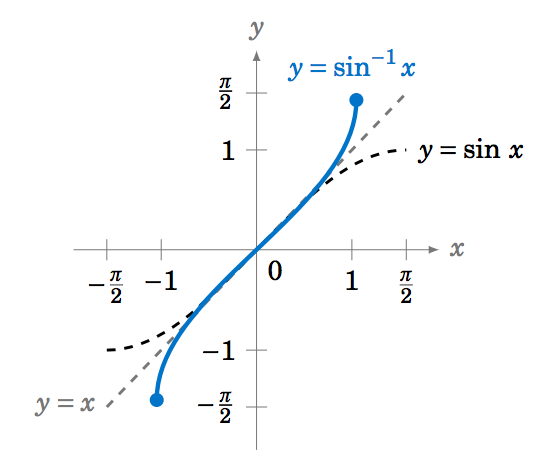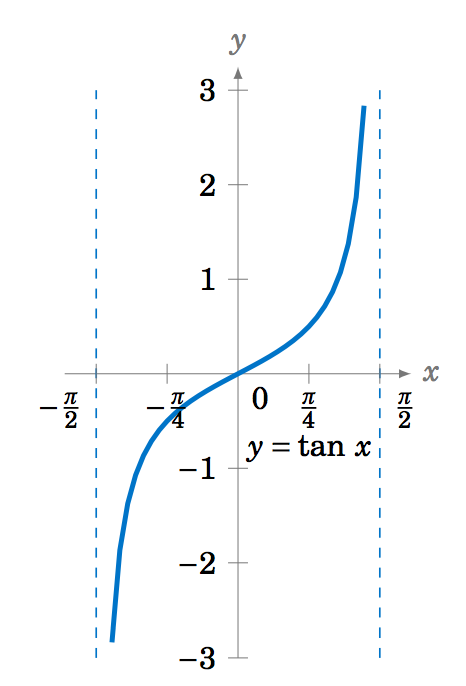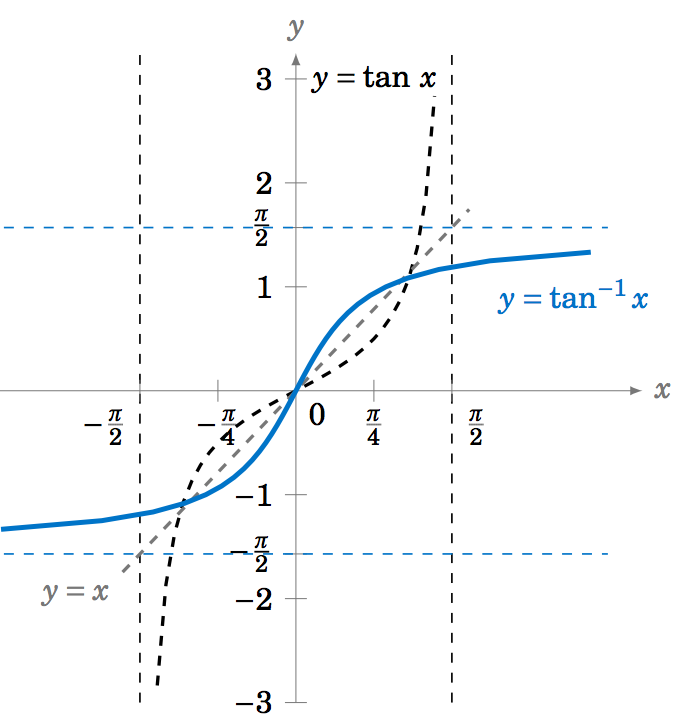$$\newcommand{\id}{\mathrm{id}}$$ $$\newcommand{\Span}{\mathrm{span}}$$ $$\newcommand{\kernel}{\mathrm{null}\,}$$ $$\newcommand{\range}{\mathrm{range}\,}$$ $$\newcommand{\RealPart}{\mathrm{Re}}$$ $$\newcommand{\ImaginaryPart}{\mathrm{Im}}$$ $$\newcommand{\Argument}{\mathrm{Arg}}$$ $$\newcommand{\norm}{\| #1 \|}$$ $$\newcommand{\inner}{\langle #1, #2 \rangle}$$ $$\newcommand{\Span}{\mathrm{span}}$$

5.3: Inverse Trigonometric Functions

•• Contributed by Michael Corral
• Professor (Mathematics) at Schoolcraft College

$$\newcommand{\vecs}{\overset { \rightharpoonup} {\mathbf{#1}} }$$

$$\newcommand{\vecd}{\overset{-\!-\!\rightharpoonup}{\vphantom{a}\smash {#1}}}$$

We have briefly mentioned the inverse trigonometric functions before, for example in Section 1.3 when we discussed how to use the $$\fbox{\(\sin^{-1}$$}\), $$\fbox{\(\cos^{-1}$$}\), and $$\fbox{\(\tan^{-1}$$}\) buttons on a calculator to find an angle that has a certain trigonometric function value. We will now define those inverse functions and determine their graphs.

Recall that a function is a rule that assigns a single object $$y$$ from one set (the range to each object $$x$$ from another set (the domain). We can write that rule as $$y = f(x)$$, where $$f$$ is the function (see Figure 5.3.1). There is a simple vertical rule for determining whether a rule $$y=f(x)$$ is a function: $$f$$ is a function if and only if every vertical line intersects the graph of $$y=f(x)$$ in the $$xy$$-coordinate plane at most once (see Figure 5.3.2).Figure 5.3.2 Vertical rule for functions

Recall that a function $$f$$ is one-to-one (often written as $$1-1$$) if it assigns distinct values of $$y$$ to distinct values of $$x$$. In other words, if $$x_1 \ne x_2$$ then $$f(x_1 ) \ne f(x_2 )$$. Equivalently, $$f$$ is one-to-one if $$f(x_1 ) = f(x_2 )$$ implies $$x_1 = x_2$$. There is a simple horizontal rule for determining whether a function $$y=f(x)$$ is one-to-one: $$f$$ is one-to-one if and only if every horizontal line intersects the graph of $$y=f(x)$$ in the $$xy$$-coordinate plane at most once (see Figure 5.3.3).Figure 5.3.3 Horizontal rule for one-to-one functions

If a function $$f$$ is one-to-one on its domain, then $$f$$ has an inverse function, denoted by $$f^{-1}$$, such that $$y=f(x)$$ if and only if $$f^{-1}(y) = x$$. The domain of $$f^{-1}$$ is the range of $$f$$.

The basic idea is that $$f^{-1}$$ "undoes'' what $$f$$ does, and vice versa. In other words,
\nonumber \begin{alignat*}{3} f^{-1}(f(x)) ~&=~ x \quad&&\text{for all $$x$$ in the domain of $$f$$, and}\\ \nonumber f(f^{-1}(y)) ~&=~ y \quad&&\text{for all $$y$$ in the range of $$f$$.} \end{alignat*}

We know from their graphs that none of the trigonometric functions are one-to-one over their entire domains. However, we can restrict those functions to subsets of their domains where they are one-to-one. For example, $$y=\sin\;x$$ is one-to-one over the interval $$\left[ -\frac{\pi}{2},\frac{\pi}{2} \right]$$, as we see in the graph below:Figure 5.3.4 $$y = \sin x$$ with $$x$$ restricted to $$\left [ − \frac{π}{ 2} , \frac{π}{ 2} \right ]$$

For $$-\frac{\pi}{2} \le x \le \frac{\pi}{2}$$ we have $$-1 \le \sin\;x \le 1$$, so we can define the inverse sine function $$y=\sin^{-1} x$$ (sometimes called the arc sine and denoted by $$y=\arcsin\;x$$) whose domain is the interval $$[-1,1]$$ and whose range is the interval $$\left[ -\frac{\pi}{2},\frac{\pi}{2} \right]$$. In other words:

\begin{alignat}{3} \sin^{-1} (\sin\;y) ~&=~ y \quad&&\text{for $$-\tfrac{\pi}{2} \le y \le \tfrac{\pi}{2}$$}\label{eqn:arcsin1}\\ \sin\;(\sin^{-1} x) ~&=~ x \quad&&\text{for $$-1 \le x \le 1$$}\label{eqn:arcsin2} \end{alignat}

Example 5.13

Find $$\sin^{-1} \left(\sin\;\frac{\pi}{4}\right)$$.

Solution:

Since $$-\frac{\pi}{2} \le \frac{\pi}{4} \le \frac{\pi}{2}$$, we know that $$\sin^{-1} \left(\sin\;\frac{\pi}{4}\right) = \boxed{\frac{\pi}{4}}\;$$, by Equation \ref{eqn:arcsin1}.

Example 5.14

Find $$\sin^{-1} \left(\sin\;\frac{5\pi}{4}\right)$$.

Solution:

Since $$\frac{5\pi}{4} > \frac{\pi}{2}$$, we can not use Equation \ref{eqn:arcsin1}. But we know that $$\sin\;\frac{5\pi}{4} = -\frac{1}{\sqrt{2}}$$. Thus, $$\sin^{-1} \left(\sin\;\frac{5\pi}{4}\right) = \sin^{-1} \left( -\frac{1}{\sqrt{2}} \right)$$ is, by definition, the angle $$y$$ such that $$-\frac{\pi}{2} \le y \le \frac{\pi}{2}$$ and $$\sin\;y = -\frac{1}{\sqrt{2}}$$. That angle is $$y=-\frac{\pi}{4}$$, since

$\sin\;\left( -\tfrac{\pi}{4} \right) ~=~ -\sin\;\left( \tfrac{\pi}{4} \right) ~=~ -\tfrac{1}{\sqrt{2}} ~. \nonumber$

Thus, $$\sin^{-1} \left(\sin\;\frac{5\pi}{4}\right) = \boxed{-\tfrac{\pi}{4}}\;$$.

Example 5.14 illustrates an important point: $$\sin^{-1} x$$ should always be a number between $$-\frac{\pi}{2}$$ and $$\frac{\pi}{2}$$. If you get a number outside that range, then you made a mistake somewhere. This why in Example 1.27 in Section 1.5 we got $$\sin^{-1}(-0.682) = -43^\circ$$ when using the $$\fbox{\(\sin^{-1}$$}\) button on a calculator. Instead of an angle between $$0^\circ$$ and $$360^\circ$$ (i.e. $$0$$ to $$2\pi$$ radians) we got an angle between $$-90^\circ$$ and $$90^\circ$$ (i.e. $$-\frac{\pi}{2}$$ to $$\frac{\pi}{2}$$ radians).

In general, the graph of an inverse function $$f^{-1}$$ is the reflection of the graph of $$f$$ around the line $$y=x$$. The graph of $$y=\sin^{-1} x$$ is shown in Figure 5.3.5. Notice the symmetry about the line $$y=x$$ with the graph of $$y=\sin\;x$$.Figure 5.3.5 Graph of $$y = \sin^{−1} x$$

The inverse cosine function $$y=\cos^{-1} x$$ (sometimes called the arc cosine and denoted by $$y=\arccos\;x$$) can be determined in a similar fashion. The function $$y=\cos\;x$$ is one-to-one over the interval $$[0,\pi]$$, as we see in the graph below:Figure 5.3.6 $$y = \cos x$$ with $$x$$ restricted to $$[0,π]$$

Thus, $$y=\cos^{-1} x$$ is a function whose domain is the interval $$[-1,1]$$ and whose range is the interval $$[0,\pi]$$. In other words:

\begin{alignat}{3} \cos^{-1} (\cos\;y) ~&=~ y \quad&&\text{for $$0 \le y \le \pi$$}\label{eqn:arccos1}\\ \cos\;(\cos^{-1} x) ~&=~ x \quad&&\text{for $$-1 \le x \le 1$$}\label{eqn:arccos2} \end{alignat}

The graph of $$y=\cos^{-1} x$$ is shown below in Figure 5.3.7. Notice the symmetry about the line $$y=x$$ with the graph of $$y=\cos\;x$$.Figure 5.3.7 Graph of $$y = \cos^{−1} x$$

Example 5.15

Find $$\cos^{-1} \left(\cos\;\frac{\pi}{3}\right)$$.

Solution:

Since $$0 \le \frac{\pi}{3} \le \pi$$, we know that $$\cos^{-1} \left(\cos\;\frac{\pi}{3}\right) = \boxed{\frac{\pi}{3}}\;$$, by Equation \ref{eqn:arccos1}.

Example 5.16

Find $$\cos^{-1} \left(\cos\;\frac{4\pi}{3}\right)$$.

Solution:

Since $$\frac{4\pi}{3} > \pi$$, we can not use Equation \ref{eqn:arccos1}. But we know that $$\cos\;\frac{4\pi}{3} = -\frac{1}{2}$$. Thus, $$\cos^{-1} \left(\cos\;\frac{4\pi}{3}\right) = \cos^{-1} \left( -\frac{1}{2} \right)$$ is, by definition, the angle $$y$$ such that $$0 \le y \le \pi$$ and $$\cos\;y = -\frac{1}{2}$$. That angle is $$y=\frac{2\pi}{3}$$ (i.e. $$120^\circ$$). Thus, $$\cos^{-1} \left(\cos\;\frac{4\pi}{3}\right) = \boxed{\tfrac{2\pi}{3}}\;$$.

Examples 5.14 and 5.16 may be confusing, since they seem to violate the general rule for inverse functions that $$f^{-1}(f(x)) = x$$ for all $$x$$ in the domain of $$f$$. But that rule only applies when the function $$f$$ is one-to-one over its entire domain. We had to restrict the sine and cosine functions to very small subsets of their entire domains in order for those functions to be one-to-one. That general rule, therefore, only holds for $$x$$ in those small subsets in the case of the inverse sine and inverse cosine.

The inverse tangent function $$y=\tan^{-1} x$$ (sometimes called the arc tangent and denoted by $$y=\arctan\;x$$) can be determined similarly. The function $$y=\tan\;x$$ is one-to-one over the interval $$\left( -\frac{\pi}{2},\frac{\pi}{2} \right)$$, as we see in Figure 5.3.8:Figure 5.3.8 $$y = \tan x \text{ with }x \text{ restricted to }\left ( − \frac{π}{ 2} , \frac{π}{ 2} \right )$$

The graph of $$y=\tan^{-1} x$$ is shown below in Figure 5.3.9. Notice that the vertical asymptotes for $$y=\tan\;x$$ become horizontal asymptotes for $$y=\tan^{-1} x$$. Note also the symmetry about the line $$y=x$$ with the graph of $$y=\tan\;x$$.Figure 5.3.9 Graph of $$y = \tan^{−1} x$$

Thus, $$y=\tan^{-1} x$$ is a function whose domain is the set of all real numbers and whose range is the interval $$\left( -\frac{\pi}{2},\frac{\pi}{2} \right)$$. In other words:

\begin{alignat}{3} \tan^{-1} (\tan\;y) ~&=~ y \quad&&\text{for $$-\tfrac{\pi}{2} < y < \tfrac{\pi}{2}$$}\label{eqn:arctan1}\\ \tan\;(\tan^{-1} x) ~&=~ x \quad&&\text{for all real $$x$$}\label{eqn:arctan2} \end{alignat}

Example 5.17

Find $$\tan^{-1} \left(\tan\;\frac{\pi}{4}\right)$$.

Solution:

Since $$-\tfrac{\pi}{2} \le \tfrac{\pi}{4} \le \tfrac{\pi}{2}$$, we know that $$\tan^{-1} \left(\tan\;\frac{\pi}{4}\right) = \boxed{\frac{\pi}{4}}\;$$, by Equation \ref{eqn:arctan1}.

Example 5.18

Find $$\tan^{-1} \left(\tan\;\pi\right)$$.

Solution:

Since $$\pi > \tfrac{\pi}{2}$$, we can not use Equation \ref{eqn:arctan1}. But we know that $$\tan\;\pi = 0$$. Thus, $$\tan^{-1} \left(\tan\;\pi\right) = \tan^{-1} 0$$ is, by definition, the angle $$y$$ such that $$-\tfrac{\pi}{2} \le y \le \tfrac{\pi}{2}$$ and $$\tan\;y = 0$$. That angle is $$y=0$$. Thus, $$\tan^{-1} \left(\tan\;\pi \right) = \boxed{0}\;$$.

Example 5.19

Find the exact value of $$\cos\;\left(\sin^{-1}\;\left(-\frac{1}{4}\right)\right)$$.

Solution:

Let $$\theta = \sin^{-1}\;\left(-\frac{1}{4}\right)$$. We know that $$-\tfrac{\pi}{2} \le \theta \le \tfrac{\pi}{2}$$, so since $$\sin\;\theta = -\frac{1}{4} < 0$$, $$\theta$$ must be in QIV. Hence $$\cos\;\theta > 0$$. Thus,

$\cos^2 \;\theta ~=~ 1 ~-~ \sin^2 \;\theta ~=~ 1 ~-~ \left( -\frac{1}{4} \right)^2 ~=~\frac{15}{16} \quad\Rightarrow\quad \cos\;\theta ~=~ \frac{\sqrt{15}}{4} ~. \nonumber$

Note that we took the positive square root above since $$\cos\;\theta > 0$$. Thus, $$\cos\;\left(\sin^{-1}\;\left(-\frac{1}{4}\right)\right) = \boxed{\frac{\sqrt{15}}{4}}\;$$.

Example 5.20

Show that $$\tan\;(\sin^{-1} x) = \dfrac{x}{\sqrt{1 - x^2}}$$ for $$-1 < x < 1$$.

Solution:

When $$x=0$$, the Equation holds trivially, since

$\nonumber \tan\;(\sin^{-1} 0) ~=~ \tan\;0 ~=~ 0 ~=~ \dfrac{0}{\sqrt{1 - 0^2}} ~.$

Now suppose that $$0 < x < 1$$. Let $$\theta = \sin^{-1} x$$. Then $$\theta$$ is in QI and $$\sin\;\theta = x$$. Draw a right triangle with an angle $$\theta$$ such that the opposite leg has length $$x$$ and the hypotenuse has length $$1$$, as in Figure 5.3.10 (note that this is possible since $$0 < x < 1$$). Then $$\sin\;\theta = \frac{x}{1} = x$$. By the Pythagorean Theorem, the adjacent leg has length $$\sqrt{1 - x^2}$$. Thus, $$\tan\;\theta = \frac{x}{\sqrt{1 - x^2}}$$.

If $$-1 < x < 0$$ then $$\theta = \sin^{-1} x$$ is in QIV. So we can draw the same triangle except that it would be "upside down'' and we would again have $$\tan\;\theta = \frac{x}{\sqrt{1 - x^2}}$$, since the tangent and sine have the same sign (negative) in QIV. Thus, $$\tan\;(\sin^{-1} x) = \dfrac{x}{\sqrt{1 - x^2}}$$ for $$-1 < x < 1$$.

The inverse functions for cotangent, cosecant, and secant can be determined by looking at their graphs. For example, the function $$y=\cot\;x$$ is one-to-one in the interval $$(0,\pi)$$, where it has a range equal to the set of all real numbers. Thus, the inverse cotangent $$y=\cot^{-1} x$$ is a function whose domain is the set of all real numbers and whose range is the interval $$(0,\pi)$$. In other words:

\begin{alignat}{3} \cot^{-1} (\cot\;y) ~&=~ y \quad&&\text{for $$0 < y < \pi$$}\label{eqn:arccot1}\\ \cot\;(\cot^{-1} x) ~&=~ x \quad&&\text{for all real $$x$$}\label{eqn:arccot2} \end{alignat}

The graph of $$y=\cot^{-1} x$$ is shown below in Figure 5.3.11.Figure 5.3.11 Graph of $$y = \cot^{−1} x$$

Similarly, it can be shown that the inverse cosecant $$y=\csc^{-1} x$$ is a function whose domain is $$|x| \ge 1$$ and whose range is $$-\frac{\pi}{2} \le y \le \frac{\pi}{2}$$, $$y \ne 0$$. Likewise, the inverse secant $$y=\sec^{-1} x$$ is a function whose domain is $$|x| \ge 1$$ and whose range is $$0 \le y \le \pi$$, $$y \ne \frac{\pi}{2}$$.

\begin{alignat}{3} \csc^{-1} (\csc\;y) ~&=~ y \quad&&\text{for $$-\frac{\pi}{2} \le y \le \frac{\pi}{2}$$, $$y \ne 0$$}\label{eqn:arccsc1}\\ \csc\;(\csc^{-1} x) ~&=~ x \quad&&\text{for $$|x| \ge 1$$}\label{eqn:arccsc2} \end{alignat}

\begin{alignat}{3} \sec^{-1} (\sec\;y) ~&=~ y \quad&&\text{for $$0 \le y \le \pi$$, $$y \ne \frac{\pi}{2}$$}\label{eqn:arcsec1}\\ \sec\;(\sec^{-1} x) ~&=~ x \quad&&\text{for $$|x| \ge 1$$}\label{eqn:arcsec2} \end{alignat}

It is also common to call $$\cot^{-1} x$$, $$\csc^{-1} x$$, and $$\sec^{-1} x$$ the arc cotangent, arc cosecant, and arc secant, respectively, of $$x$$. The graphs of $$y=\csc^{-1} x$$ and $$y=\sec^{-1} x$$ are shown in Figure 5.3.12:

Example 5.21

Prove the identity $$\tan^{-1} x \;+\; \cot^{-1} x ~=~ \frac{\pi}{2}$$.

Solution:

Let $$\theta = \cot^{-1} x$$. Using relations from Section 1.5, we have

$\nonumber \tan\;\left( \tfrac{\pi}{2} - \theta \right) ~=~ -\tan\;\left( \theta - \tfrac{\pi}{2} \right) ~=~ \cot\;\theta ~=~ \cot\;(\cot^{-1} x) ~=~ x ~,$

by Equation \ref{eqn:arccot2}. So since $$\tan\;(\tan^{-1} x) = x$$ for all $$x$$, this means that $$\tan\;(\tan^{-1} x) = \tan\;\left( \tfrac{\pi}{2} - \theta \right)$$. Thus, $$\tan\;(\tan^{-1} x) = \tan\;\left( \tfrac{\pi}{2} - \cot^{-1} x \right)$$. Now, we know that $$0 < \cot^{-1} x < \pi$$, so $$-\tfrac{\pi}{2} < \tfrac{\pi}{2} - \cot^{-1} x < \tfrac{\pi}{2}$$, i.e. $$\tfrac{\pi}{2} - \cot^{-1} x$$ is in the restricted subset on which the tangent function is one-to-one. Hence, $$\tan\;(\tan^{-1} x) = \tan\;\left( \tfrac{\pi}{2} - \cot^{-1} x \right)$$ implies that $$\tan^{-1} x = \tfrac{\pi}{2} - \cot^{-1} x$$, which proves the identity.

Example 5.22

Is $$\;\tan^{-1} a \;+\; \tan^{-1} b ~=~ \tan^{-1} \left( \dfrac{a+b}{1-ab} \right)\;$$ an identity?

Solution:

In the tangent addition Equation $$\tan\;(A+B) = \dfrac{\tan\;A \;+\; \tan\;B}{1 \;-\; \tan\;A~\tan\;B}$$, let $$A = \tan^{-1} a$$ and $$B = \tan^{-1} b$$. Then

\nonumber \begin{align*} \tan\;(\tan^{-1} a \;+\; \tan^{-1} b ) ~&=~ \dfrac{\tan\;(\tan^{-1} a) \;+\; \tan\;(\tan^{-1} b)}{1 \;-\; \tan\;(\tan^{-1} a)~\tan\;(\tan^{-1} b)}\\ \nonumber &=~ \dfrac{a+b}{1-ab}\qquad\text{by Equation \ref{eqn:arctan2}, so it seems that we have}\\ \nonumber \tan^{-1} a \;+\; \tan^{-1} b ~&=~ \tan^{-1} \left( \dfrac{a+b}{1-ab} \right) \end{align*}

by definition of the inverse tangent. However, recall that $$-\tfrac{\pi}{2} < \tan^{-1} x < \tfrac{\pi}{2}$$ for all real numbers $$x$$. So in particular, we must have $$-\tfrac{\pi}{2} < \tan^{-1} \left( \frac{a+b}{1-ab} \right) < \tfrac{\pi}{2}$$. But it is possible that $$\tan^{-1} a \;+\; \tan^{-1} b$$ is not in the interval $$\left(-\tfrac{\pi}{2},\tfrac{\pi}{2}\right)$$. For example,

$\tan^{-1} 1 \;+\; \tan^{-1} 2 ~=~ 1.892547 ~>~ \tfrac{\pi}{2} \approx 1.570796 ~.\nonumber$

And we see that $$\tan^{-1} \left( \frac{1+2}{1-(1)(2)} \right) = \tan^{-1} (-3) = -1.249045 \ne \tan^{-1} 1 \;+\; \tan^{-1} 2$$. So the Equation is only true when $$-\tfrac{\pi}{2} < \tan^{-1} a \;+\; \tan^{-1} b < \tfrac{\pi}{2}$$.